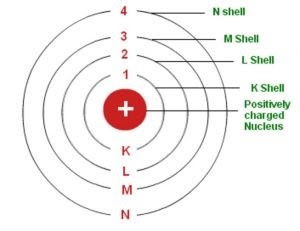# Bohr Model of the Hydrogen Atom, Postulate | Limitation

Postulates, Limitation, Bohr made certain modifications in Rutherford's atom model by adding the ideas of newly developing quantum hypothesis.

## Bohr Model

In 1913, Niels Bohr made certain modifications in Rutherford's atom model by adding the ideas of newly developing quantum hypothesis. Bohr argued that although classical electromagnetic theory is extremely successful in explanation phenomena at macroscale but it needs a radial modification to explain the structure of atoms and relation of atomic structure to atomic spectra.

Bohr combined classical and early quantum, concepts and proposed a modified nuclear model of atom, nowadays know as Bohr's atom model.

## Postulates of Bohr Atom Model

Bohr atom model is based on the following three postulates:

(1) An atom consists of a tiny, central part called nucleus, in which whole positive charge and almost whole mass of an atom is concentrated. The electron revolves around the nucleus in certain specified circular orbits without the emission of radiant energy. These orbits are called stable orbits.Bohr Atom Model

The first postulate of Bohr signifies that each atom has certain definite stable states in which it can exist and each possible state has definite total energy. These states are called the stationary states of the atom.

(2) Bohr's quantum condition:- Bohr's second postulate defines the stable orbits. It states that the electron revolves around the nucleus only in those orbits for which its angular momentum is some integer multiple of h/2π, where h is the planck's constant. i.e.

L = mvnrn = nh/2π, where n = 1,2,3,4,...

The number n is generally known as the principal quantum member. The second postulate signifies the quantization of angular momentum.

(3) Quantum concept of radiant energy:- It states that whenever an electron makes a transition from one stable specified non-radiating orbit, it emits/absorbs a radiation photon whose energy is equal to the energy difference between the initial and final states.Bohr Atom Model

\Delta E=E_i-E_f=h\nu=\frac{hc}\lambda

Where, Ei = Energy of initial states

Ef = Energy of final states

If Ei > Ef, then a photon is emitted in the process. However, if Ei < Ef, then the electron must absorb a photon to go to higher energy state.

Thus, we can see that as per Bohr atom model, the various lines in atomic spectra of hydrogen are produced when electrons jump from higher energy state to lower energy state and photons of appropriate energy or frequency are emitted. These spectral lines are called emission lines.

## Limitations of Bohr Atom Model

Although Bohr atom model correctly predicts the gross features, specially the frequency/wavelength of radiation emitted or selectively absorbed, yet the model has many limitations. Some of important limitations are as follows:

1. The Bohr model is applicable to hydrogen and hydrogenic atoms only. It cannot be extended for atoms with more than one electron.

2. Bohr atom model fails to explain the fine structure of the spectral lines.

3. Bohr's theory fails to account for the variations of intensities of the frequencies/wavelengths in the spectrum.

4. There is no logical explanation of considering the electron orbits only as circular orbits. Elliptical orbits are also possible.

5. Bohr atom model fails to explain Zeeman effect (magnetic effects on atomic spectra) and Stark effect (electrical effects on atomic spectra).